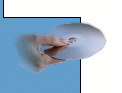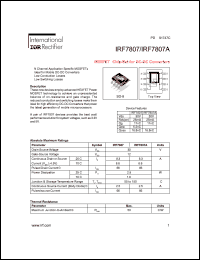More than4 307 195 components listedIRF7807 series datasheets. Manufacturer: International Rectifier.

 IRF7807 HEXFET chip-set for DC-DC converters. VDSS = 30V. RDS(on) = 25mOhm. in 8-pin SO package. Operational temperature range from -55°C to 150°C. Datasheet*) IRF7807A HEXFET chip-set for DC-DC converters. VDSS = 30V. RDS(on) = 25mOhm. in 8-pin SO package. Operational temperature range from -55°C to 150°C. Datasheet*) IRF7807D1 FETKY MOSFET and schottky diode. VDS = 30V, RDS(on) =25mOhm in 8-pin SO package. Operational temperature range from -55°C to 150°C. Datasheet*) IRF7807D2 FETKY MOSFET and schottky diode. VDS = 30V, RDS(on) =25mOhm in 8-pin SO package. Operational temperature range from -55°C to 150°C. Datasheet*) IRF7807V FETKY MOSFET and schottky diode. VDS = 30V, RDS(on) =17mOhm in 8-pin SO package. Operational temperature range from -55°C to 150°C. Datasheet*) IRF7807VD1 FETKY MOSFET and schottky diode. VDS = 30V, RDS(on) =17mOhm in 8-pin SO package. Operational temperature range from -55°C to 150°C. Datasheet*) IRF7807VD2 FETKY MOSFET and schottky diode. VDS = 30V, RDS(on) =17mOhm in 8-pin SO package. Operational temperature range from -55°C to 150°C. Datasheet*)